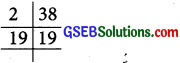# GSEB Solutions Class 6 Maths Chapter 3 Playing With Numbers InText Questions

Gujarat Board GSEB Textbook Solutions Class 6 Maths Chapter 3 Playing With Numbers InText Questions and Answers.

## Gujarat Board Textbook Solutions Class 6 Maths Chapter 3 Playing With Numbers InText Questions

Try These (Page 48)

Question 1.
Find the possible factors of 45, 30 and 36.
Solution:
(i) Factors of 45:
We have: 45 = 1 x 45
45 = 3 x 15
45 = 5 x 9
Factors of 45 are: 1, 3, 5, 9, 15 and 45.

(ii) Factors of 30:
30 = 1 x 30
30 = 2 x 15
30 = 3 x 10
30 = 5 x 6
Factors of 30 are: 1,2,3, 5,6, 10, 15 and 30.

(iii) Factors of 36:
36 = 1 x 36
36 = 2 x 18
36 = 3 x 12
36 = 4 x 9
36 = 6 x 6
Factors of 36 are: 1,2,3,4, 6,9, 12, 18 and 36.Try These (Page 52)

Question 1.
Observe that 2 x 3 + 1 = 7 is a prime number Here,
I has been added to a multiple of 2 to get a prime number.
Can you find some more numbers of this type?
Solution:
We can have:
2 x 2 + 1 = 5, which is a prime number.
2 x 5 + 1 = 11, which is a prime number.
2 x 6 + 1 = 13, which is a prime number.
2 x 8 + 1 = 17, which is a prime number.
2 x 9 + 1 = 19, which iš a prime number.
2 x 10 + 1 = 23, which is a prime number.

Try These (Page 58)

Question 1.
Find the common factors of
(a) 8, 20
(b) 9, 15
Solution:
(a) 8, 20
Since,
8 = 1 x 8
8 = 2 x 4
All the factors of 8 are: 1, 2, 4 and 8 ………….(i)
Again,
20 = 1 x 20
20 = 2 x 10
20 = 4 x 5
All the factors of 20 are: 1, 2, 4, 5, 10 and 20 ……………(ii)
From
(i) and
(ii), common factors of 8 and 20 are: 1, 2 and 4.

(b) 9, 15
Wehave :
9 = 1 x 9
9 = 3 x 3
All the factors of 9 are: 1, 3 and 9 ………….(i)
Again, 15 = 1 x 15
15 = 3 x 5
All the factors of 15 are: 1, 3, 5 and 15
From
(i) and
(ii), common factors of 9 and 15 are: 1 and 3.Try These (Page 61)

Question 1.
Write the prime factorisations of 16, 28, 38.
Solution:
(i) 16
We have:Prime factorisationof 16 = 2 x 2 x 2 x 2

(ii) 28
We have:Prime factorisation of 28 = 2 x 2 x 7

(iii) 38
We have:Prime factorisation of 38 = 2 x 19Try These (Page 63)

Question 1.
Find the HCF of the following:
(i) 24 and 36
(ii) 15, 25 and 30
(iii) 8 and 12
(iv) 12, 16 and 28
Solution:
(i) 24 and 36
Factors of 24 are: 1, 2, 3, 4, 6, 12 and 24
Factors of 36 are: 1, 2,3,4,6,9, 12, 18 and 36
Common factors are: 1, 2, 3, 4, 6 and 12
Since, the highest of these common factors = 12
HCF of 24 and 36 = 12

(ii) 15, 25 and 30
Factors of 15 are: 1, 3, 5 and 15
Factors of 25 are: 1, 5 and 25
Factors of 30 are: 1, 2, 3, 5, 6, 10, 15 and 30
Common factors are: 1 and 5
Since, the highest common factor is 5,
HCF of 15, 25 and 30 = 5

(iii) 8 and 12
Factors of 8 are: 1, 2, 4 and 8
Factors of 12 are: 1, 2, 3, 4, 6 and 12
Common factors are: 1, 2, 4
Since, the highest common factor is 4,
HCF of 8 and 12 = 4

(iv) 12, 16 and 28
Factors of 12 are: 1, 2, 3, 4, 6 and 12
Factors of 16 are: 1, 2, 4, 8 and 16
Factors of 28 are: 1, 2,4, 7, 14 and 28
Common factors are: 1, 2, 4
Since, the highest common factor = 4,
HCF of 12, 16 and 28 = 4